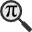## General

Display information for equation id:math.7622.11 on revision:7622

* Page found: Abbe sine condition (eq math.7622.11)

(force rerendering)

Cannot find the equation data in the database. Fetching from revision text.

Occurrences on the following pages:

Hash: c3caaffa8f70272e461c725e3ae50211

TeX (original user input):

\phi =0


TeX (checked):

\phi =0


### LaTeXML (experimental; uses MathML) rendering

MathML (871 B / 340 B) :${\displaystyle\phi=0}$
<math xmlns="http://www.w3.org/1998/Math/MathML" id="p1.1.m1.1" class="ltx_Math" alttext="{\displaystyle\phi=0}" display="inline">
<semantics id="p1.1.m1.1a">
<mrow id="p1.1.m1.1.4" xref="p1.1.m1.1.4.cmml">
<mi id="p1.1.m1.1.1" xref="p1.1.m1.1.1.cmml">ϕ</mi>
<mo id="p1.1.m1.1.2" xref="p1.1.m1.1.2.cmml">=</mo>
<mn id="p1.1.m1.1.3" xref="p1.1.m1.1.3.cmml">0</mn>
</mrow>
<annotation-xml encoding="MathML-Content" id="p1.1.m1.1b">
<apply id="p1.1.m1.1.4.cmml" xref="p1.1.m1.1.4">
<eq id="p1.1.m1.1.2.cmml" xref="p1.1.m1.1.2"/>
<ci id="p1.1.m1.1.1.cmml" xref="p1.1.m1.1.1">italic-ϕ</ci>
<cn type="integer" id="p1.1.m1.1.3.cmml" xref="p1.1.m1.1.3">0</cn>
</apply>
</annotation-xml>
<annotation encoding="application/x-tex" id="p1.1.m1.1c">{\displaystyle\phi=0}</annotation>
</semantics>
[/itex]


SVG (2.182 KB / 1.064 KB) :

### MathML with SVG or PNG fallback (recommended for modern browsers and accessibility tools) rendering

SVG image empty. Force Re-Rendering

SVG (0 B / 8 B) :

PNG (0 B / 8 B) :

$\phi =0$## Translations to Computer Algebra Systems

### Translation to Maple

In Maple: phi = 0

\phi: Could be the golden ratio == golden mean == golden section == extreme and mean ratio == medial section == divine proportion == divine section == golden proportion == golden cut == golden number.

But this system don't know how to translate it as a constant. It was translated as a general letter.


### Translation to Mathematica

In Mathematica: \[Phi]= 0

\phi: Could be the golden ratio == golden mean == golden section == extreme and mean ratio == medial section == divine proportion == divine section == golden proportion == golden cut == golden number.

But this system don't know how to translate it as a constant. It was translated as a general letter.


## Similar pages

Calculated based on the variables occurring on the entire Abbe sine condition page

## Identifiers

• $\phi$### MathML observations

0results

0results

no statistics present please run the maintenance script ExtractFeatures.php

0 results

0 results